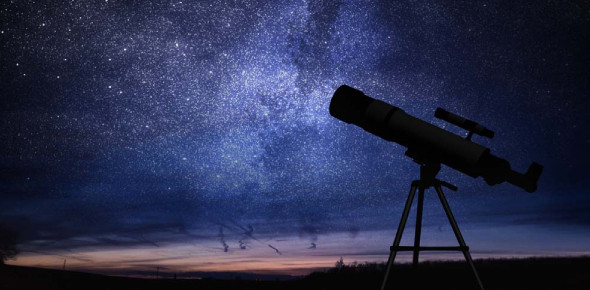# Astronomy Test: Basic Questions! Quiz

21 Questions | Total Attempts: 108SettingsHow much do you know about astronomy? Can you take a shot at this quiz? Astronomy is the science that studies the stars, planets, moons, galaxies, and comets. It focuses on natural phenomena. It depends upon mathematics, physics, and chemistry to describe their origin and evolution. Astronomy is concerned with celestial bodies that reside in outer space. This quiz is incredible, and your participation is written in the stars.

• 1.
The sun gives us three things that we need for life on earth. What are they?
• A.

Light, energy and daytime

• B.

Light, heat and warmth

• C.

Light, heat and energy

• D.

Light, heat and mornings

• E.

None of these are correct

• 2.
What is one important thing that we get from the sun?
• 3.
The sun gives us heat.
• A.

False

• B.

True

• 4.
The sun is located in the _____________ of the solar system.
• A.

Top

• B.

Bottom

• C.

Center

• D.

End

• E.

Beginning

• 5.
The sun is not located in the center of the solar system.
• A.

False

• B.

True

• 6.
How long does it take for the earth to rotate one time?
• 7.
It takes one month for the Earth to rotate on its axis.
• A.

False

• B.

True

• 8.
The sun revolves around the Earth.
• A.

False

• B.

True

• 9.
The Earth orbits the sun.
• A.

False

• B.

True

• 10.
A _______ Eclipse occurs when the moon lines up exactly between the sun and the Earth.
• 11.
When the sun, Earth, and moon exactly line up it is called a ________ Eclipse.
• A.

Lunar

• B.

Solar

• 12.
When it is day on one side of the earth, it is __________ on the other.
• 13.
It can be daytime on the whole Earth at the same time.
• A.

True

• B.

False

• 14.
__________  is the planet closest to the sun.
• 15.
Which are inner planets?
• A.

Saturn

• B.

Venus

• C.

Earth

• D.

Mars

• E.

Jupiter

• 16.
Which are outer planets?
• A.

Mars

• B.

Saturn

• C.

Jupiter

• D.

Pluto

• 17.
A group of stars formed into patterns is called a ______________
• A.

Galaxy

• B.

Star system

• C.

Constellation

• D.

Solar system

• E.

Universe

• 18.
Neptune is an outer planet.
• A.

False

• B.

True

• 19.
Uranus is the planet closest to the sun.
• A.

False

• B.

True

• 20.
Earth is an inner planet.
• A.

False

• B.

True

• 21.
What does the moon orbit?
• A.

The sun

• B.

The Earth

• C.

Nothing

• D.

The solar system

Related TopicsBack to top